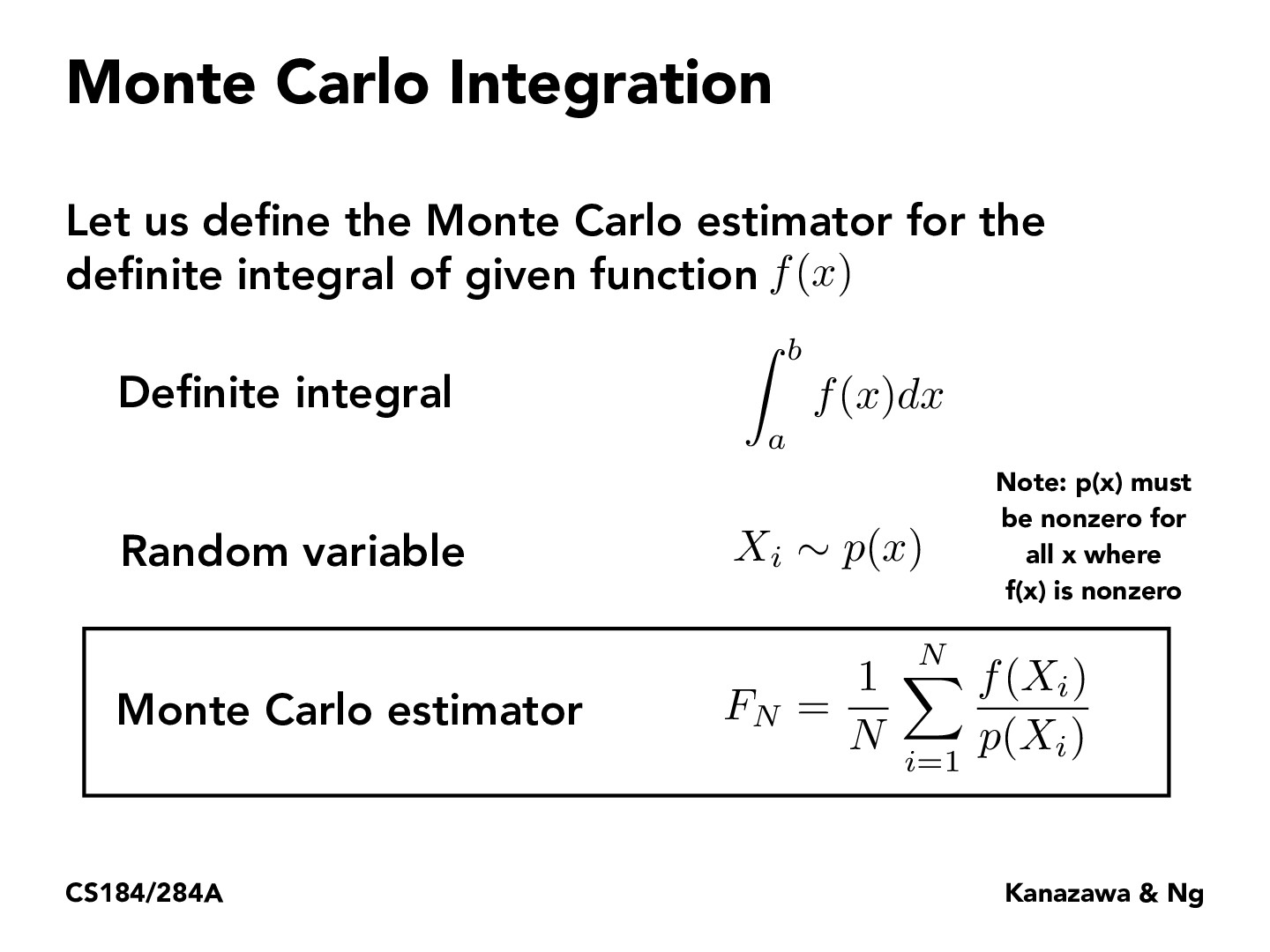Lecture 12: Monte Carlo Integration (21)han20192019

It's very important that we should normalize p(x) so that the sum of p(x) will become 1(because sum of probability is 1). And also we should be careful that p(x) should be nonzero because each probability is between 0 and 1.aravind00r

I think a really good note that Ren mentioned in the lecture was why we are dividing by P(Xi). For importance sampling specifically, since we will be taking most of our samples from the light area and not from sections where there is little contributions, our estimation will be much brighter overall than reality if we do not weight each sample by the probability function at that position.

You must be enrolled in the course to comment